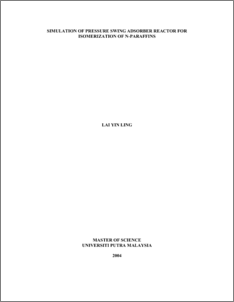# Simulation of Pressure Swing Adsorber Reactor for Isomerization of N-Paraffins

## Citation

Lai, Yin Ling (2004) Simulation of Pressure Swing Adsorber Reactor for Isomerization of N-Paraffins. Masters thesis, Universiti Putra Malaysia.

## Abstract

In this thesis, a non-isothermal pressure swing adsorber-reactor (PSAR) model for the isomerization of n-paraffins is developed. PSAR is a new concept that combines the isomerization of normal paraffins to iso-paraffins and separation of the iso-paraffins from mixtures of n/iso-paraffins by using pressure swing adsorption (PSA). The isomerization of normal-pentane (n-C5) to iso-pentane (i-C5) is used to illustrate the concept of PSAR. The PSAR column is packed with a layer of catalyst (Y-zeolite) and followed by a layer of adsorbent (zeolite 5A). The mathematical model is solved numerically using the method of lines where the partial differential equations (PDEs) are partially discretized and reduced to ordinary differential equations (ODEs).Orthogonal collocation (OC) method is used as the discretization method.The computer programme is written in FORTRAN 99. The numerical implementation of the developed PSAR is verified by adjusting the ratio of the length of the reactor section to the total length of the PSAR columnWhen approaches 0, the PSAR model is reduced to PSA separation model. When approaches 1,the model is reduced back to steady state reactor model. The optimum value of is found to be 0.6. The yield of the desired product increases by about 13 % with the optimum value of = 0.6 compared to the conventional isomerization reactor.The appropriate thermal operating condition for the PSAR is isothermal condition. To approach the isothermal operating condition, the heat transfer in the PSA section is critical because it determines the uptake of the unreacted reactant in the reaction/adsorption step and the temperature of the effluent at the end of the PSA section in the desorption/reaction step.The developed non-isothermal PSAR model is therefore useful for the design, scale-up, and control and optimization purposesPreview
PDF
549500_t_fk_2004_52.pdfView Item